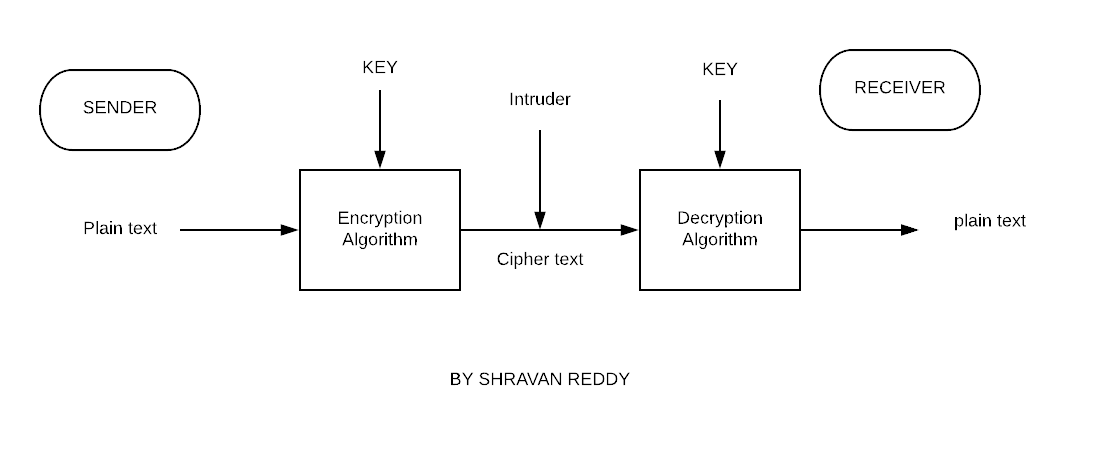# Encryption and Decryption of String using Python

In this post, we will learn a very interesting concept from cryptography that is Encryption and Decryption of strings using Python. Now what are this Encryption and Decryption, Why should you know about them? Well,
these are very interesting and are used for security purposes.

## What is meant by encryption and decryption of a string

Encryption: It is the process of converting a plain text string into a ciphertext. Now, What is this ciphertext it is nothing but the encrypted text of your given message it is non-understandable but can be only understood after decrypting.

Decryption: It is the reverse process of encryption so the ciphertext will be converted back to plaintext using the same key which we used earlier or maybe a different one.

Now let us see simple encryption and decryption example and then go for the final implementation
Example1:
Input
Enter the string to encrypt and decrypt: Shravan
Enter the Key: 5

output:
Encrypted String: Xmwfafs
Decrypted String: Shravan

Here I am changing the values of alphabets according to the key that is the first alphabet in the string is “S” so the value is shifted by 5 places from S that is “T”, “U”, “V”, “W”, “X”  so the output is “X”.
Similarly, the same process is applied on the whole string if you get to the end of the string then you must start from the beginning again.

let string be “zap” and the key is 1 then “z” is replaced by “a”.## Encryption and Decryption of a string Implementation in Python

```def Encryption(s,k):
encstr=""
for i in s:
if(ord(i))>=65 and (ord(i)<=90):
temp=(ord(i)+k)
if temp>90:
temp=temp%90+64
encstr=encstr+chr(temp)
elif(ord(i))>=97 and (ord(i)<=122):
temp=(ord(i)+k)
if temp>122:
temp=temp%122+96
encstr=encstr+chr(temp)
else:
encstr=encstr+chr(ord(i)+k)
return encstr
def Decryption(k):
p=Encryption(s,k)
decstr=""
for i in p:
if((ord(i))>=65) and (ord(i))<=90:
decstr=decstr+chr((ord(i) - k-65) % 26 + 65)
elif((ord(i))>=97) and (ord(i))<=122:
decstr=decstr+chr((ord(i) - k - 97) % 26 + 97)
else:
decstr=decstr+chr(ord(i)-k)
return decstr
print("Enter the string to Encrypt and decrypt : ")
s=input()
print("Enter the key(Eg: 21) : ")
k=int(input())
k=k%26
print("Encrypted String : ",Encryption(s,k))
print("Decrypted String : ",Decryption(k))

```
```output:
Enter the string to Encrypt and decrypt :

shravan

Enter the key(Eg: 21) :

15

Encrypted String :  hwgpkpc

Decrypted String :  shravan```

### Understanding the Implementation

Here is the code for Encryption and Decryption using Python programming language.
In the above code, there are two functions Encryption() and Decryption() we will call them by passing parameters.
the Encryption() function takes two parameters the string and the key to encrypt while the other Decryption function takes the key to decrypt the encrypted string.

In Encryption ord() function is used to find the ASCII value of the given character.
we should know what are these ASCII values in order to encrypt and decrypt string the values start
from a to z and A to Z.

“a” has the ASCII value of 97, “b” has the value of 98 and so on till “z” having value as 122.  As there are 26 alphabets.

“A” starts with the value 65, “B” has the value of 66 and so on till “Z” having value as 90.

In the code, we will check if the character is uppercase() or lowercase() using ASCII values and then if it
lies in the uppercase values we will rotate it only in uppercase alphabets. And if it is in lowercase() then we
try to rotate only in the lowercase() only.

The keyword chr() is used to convert ASCII value to char. it is stored in a new string variable.
The % operator is to find the remainder.

#### Logic behind this program

The logic is that we will go through each character of the string and find if the character is uppercase or
lowercase and then suppose if it is uppercase(lies in the range of 65-90) then we shift the characters
according to the key and if it exceeds the 90 then we should bring it back from 65. Similarly the same is the
case with decryption but we subtract or shift it backward.

I hope you have understood the code if still, you have any doubts regarding the program feel free to
comment down below.

More interesting programs are below if interested check out them.

### One response to “Encryption and Decryption of String using Python”

1. Aaron Franklin says:

Hello and thank you for this article. I am interested in how one would go about getting accurate encryption of “?” “:” and other such characters. I am working on a program that encrypts a custom string of characters. Including “+” and “&” and possibly “@” “_” etc. If you have any suggestions I would appreciate the input.